xMagnetic pole strengthEncyclopedia
Magnetic pole strength (symbol: p) is a physical quantity
Physical quantity
A physical quantity is a physical property of a phenomenon, body, or substance, that can be quantified by measurement.-Definition of a physical quantity:Formally, the International Vocabulary of Metrology, 3rd edition defines quantity as:...

used to measure the strength of the pole
Magnetic pole
Magnetic pole may refer to:* One of the two ends of a magnet* The magnetic poles of astronomical bodies, a special case of magnets, two special cases of which are the Geomagnetic poles:...

of a bar magnet (or a hypothetical magnetic monopole
Magnetic monopole
A magnetic monopole is a hypothetical particle in particle physics that is a magnet with only one magnetic pole . In more technical terms, a magnetic monopole would have a net "magnetic charge". Modern interest in the concept stems from particle theories, notably the grand unified and superstring...

). If there is an infinitely long wire where the electric current
Electric current
Electric current is a flow of electric charge through a medium.This charge is typically carried by moving electrons in a conductor such as wire...

is I, then the magnetic pole strength is defined as follows: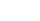,
where W is the work which has been made when the magnetic pole has been turned around the wire in a closed loop. In the definition, it has also been determined that p > 0 for the north magnetic pole
North Magnetic Pole
The Earth's North Magnetic Pole is the point on the surface of the Northern Hemisphere at which the Earth's magnetic field points vertically downwards . Though geographically in the north, it is, by the direction of the magnetic field lines, physically the south pole of the Earth's magnetic field...

and p < 0 for the south magnetic pole
South Magnetic Pole
The Earth's South Magnetic Pole is the wandering point on the Earth's surface where the geomagnetic field lines are directed vertically upwards...

.

The Ampere law follows almost immediately from the definition. The notion of magnetic pole strength may seem artificial, but when it is defined as above, it is perfectly analogous to the electric charge
Electric charge
Electric charge is a physical property of matter that causes it to experience a force when near other electrically charged matter. Electric charge comes in two types, called positive and negative. Two positively charged substances, or objects, experience a mutual repulsive force, as do two...

. Thus the magnetic field strength is defined just as the electric field strength: as the force with which the field affects to a unit north pole: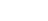.

In the International system of units (SI
Si
Si, si, or SI may refer to :- Measurement, mathematics and science :* International System of Units , the modern international standard version of the metric system...

) there is no official standard unit for magnetic pole strength, but the unit of magnetic flux
Magnetic flux
Magnetic flux , is a measure of the amount of magnetic B field passing through a given surface . The SI unit of magnetic flux is the weber...

, 1 weber
Weber
Weber is a surname of German origin, derived from the noun meaning "weaver". In some cases, following migration to English-speaking countries, it has been anglicised to the English surname 'Webber' or even 'Weaver'.Notable people with the surname include:...

(Wb) = 1 J
Joule
The joule ; symbol J) is a derived unit of energy or work in the International System of Units. It is equal to the energy expended in applying a force of one newton through a distance of one metre , or in passing an electric current of one ampere through a resistance of one ohm for one second...

/A
Ampere
The ampere , often shortened to amp, is the SI unit of electric current and is one of the seven SI base units. It is named after André-Marie Ampère , French mathematician and physicist, considered the father of electrodynamics...

= 1 V
Volt
The volt is the SI derived unit for electric potential, electric potential difference, and electromotive force. The volt is named in honor of the Italian physicist Alessandro Volta , who invented the voltaic pile, possibly the first chemical battery.- Definition :A single volt is defined as the...

s
Second
The second is a unit of measurement of time, and is the International System of Units base unit of time. It may be measured using a clock....

, can also be used for magnetic field strength. The magnetic flux around the magnetic pole is equal to the magnetic pole strength, just as the electric flux around a charge is equal to the charge.

Alternatively the magnetic pole strength is sometimes defined as the magnetic moment
Magnetic moment
The magnetic moment of a magnet is a quantity that determines the force that the magnet can exert on electric currents and the torque that a magnetic field will exert on it...

of a magnet divided by the distance of its poles. In this case, its unit us one ampere
Ampere
The ampere , often shortened to amp, is the SI unit of electric current and is one of the seven SI base units. It is named after André-Marie Ampère , French mathematician and physicist, considered the father of electrodynamics...

metre
Metre
The metre , symbol m, is the base unit of length in the International System of Units . Originally intended to be one ten-millionth of the distance from the Earth's equator to the North Pole , its definition has been periodically refined to reflect growing knowledge of metrology...

(1 Am). This quantity has not the same dimension
Dimensional analysis
In physics and all science, dimensional analysis is a tool to find or check relations among physical quantities by using their dimensions. The dimension of a physical quantity is the combination of the basic physical dimensions which describe it; for example, speed has the dimension length per...

as the one defined earlier, but their ratio is constant and equal to the universal magnetic constant,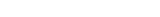.

Despite intensive research, no magnetic monopole
Magnetic monopole
A magnetic monopole is a hypothetical particle in particle physics that is a magnet with only one magnetic pole . In more technical terms, a magnetic monopole would have a net "magnetic charge". Modern interest in the concept stems from particle theories, notably the grand unified and superstring...

s have hitherto been found, but in the modern particle physics
Particle physics
Particle physics is a branch of physics that studies the existence and interactions of particles that are the constituents of what is usually referred to as matter or radiation. In current understanding, particles are excitations of quantum fields and interact following their dynamics...

such particles are considered to be theoretically possible. A bar magnet, however, especially if it is long and narrow (for example the needle of a compass
Compass
A compass is a navigational instrument that shows directions in a frame of reference that is stationary relative to the surface of the earth. The frame of reference defines the four cardinal directions – north, south, east, and west. Intermediate directions are also defined...

), behaves very much as if it were composed of two magnetic monopoles being in both ends of the bars, having equal strength but opposite sign.

## Magnetic Coulomb's law

A bar magnet can be analyzed as if it where composed of two magnetic poles of opposite sign, being at its opposite ends. In this case it turns out that the poles of two distinct magnets affect to each other with a force being directly proportional to the product of pole strengths and inversely proportional to the square of their distance. This so called magnetic Coulomb's law is exactly analogous to the electrical Coulomb's law
Coulomb's law
Coulomb's law or Coulomb's inverse-square law, is a law of physics describing the electrostatic interaction between electrically charged particles. It was first published in 1785 by French physicist Charles Augustin de Coulomb and was essential to the development of the theory of electromagnetism...

, and it can be written as a formula: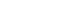where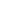is a constant. Formerly the cgs system of units was very much used in physics. In it, the electromagnetic units were defined so that this constant was one. Thus the unit of magnetic pole strength and of magnetic flux, one maxwell
Maxwell (unit)
The maxwell, abbreviated as Mx, is the compound derived CGS unit of magnetic flux. The unit was previously called a line. The unit name honours James Clerk Maxwell, who presented the unified theory of electromagnetism, and was established by the IEC in 1930.In a magnetic field of strength one...

, was equal to 10−8 Wb. Such a magnetic pole affects on another equal pole, being at the distance of one centimeter, with the force of one dyne
Dyne
In physics, the dyne is a unit of force specified in the centimetre-gram-second system of units, a predecessor of the modern SI. One dyne is equal to exactly 10 µN...

.

In current SI
Si
Si, si, or SI may refer to :- Measurement, mathematics and science :* International System of Units , the modern international standard version of the metric system...

units, the constant k must be expressed by using the magnetic constant or the permeability
Permeability (electromagnetism)
In electromagnetism, permeability is the measure of the ability of a material to support the formation of a magnetic field within itself. In other words, it is the degree of magnetization that a material obtains in response to an applied magnetic field. Magnetic permeability is typically...

of vacuum, μ0 avulla. It follows from the definition of the ampere
Ampere
The ampere , often shortened to amp, is the SI unit of electric current and is one of the seven SI base units. It is named after André-Marie Ampère , French mathematician and physicist, considered the father of electrodynamics...

that this constant is equal to 4 π · 10−7 Vs/Am. If the weber (or the volt second) is used as the unit of magnetic pole strength, the coefficient k in the formula above is equal to 1 / (4 π · μ0). If the unit ampere meter is used instead, the coefficient is μ0 / (4 π), which is exactly 107 Vs/Am.

The magnetic Coulomb's law has mainly science-historical significance. Its relevance is limited by the fact that in most magnets, the location of poles cannot be precisely specified, and therefore magnetic pole strength cannot be measured with good precision. For this reason, magnetic moment
Magnetic moment
The magnetic moment of a magnet is a quantity that determines the force that the magnet can exert on electric currents and the torque that a magnetic field will exert on it...

has turned out to be a much more useful quantity and it can be measured more accurately. If, however, magnetic monopoles turned out to exist, then, according to theory, they would obey precisely this law.